# Hybrid Diagnosis Method Applied to Switched Mechatronic Systems

Hybrid Diagnosis Method Applied to Switched Mechatronic Systems
Laboratory of Industrial Techniques (LTI), University Sidi Mohamed Ben Abdellah, Fez 30000, Morocco

Corresponding Author Email:
ayman1abboudi@gmail.com
Page:
683-691
|
DOI:
https://doi.org/10.18280/jesa.540503
20 August 2021
|
Accepted:
25 September 2021
|
Published:
31 October 2021
| Citation

OPEN ACCESS

Abstract:

This article proposes a new diagnosis approach extended to switched mechatronic systems. The best tools of modeling and supervision, notably bond graph and observer, are used to move towards a high reliable fault detection and isolation approach. Researchers have always divided the hybrid observer into two blocks: a location observer that identifies the current mode and a continuous observer that detects faults. Applying the same logic to a system with a higher number of parameters from different energy domains increases the number of calculations and leads to a combinatorial explosion. The innovative interest of the present paper is the optimization of the observer's number using only one block to detect and, at the same time, locate faults. As a second objective, this paper presents an extension of the method to include complex industrial devices, which are in most cases switched mechatronic systems.

Keywords:

diagnosis, mechatronic systems, switched systems, hybrid observer, bond graph, hybrid automaton

1. Introduction

Today’s industrial systems are of ever-increasing complexity. They exhibit hybrid dynamical behavior in various energy domains. More mechatronic systems are equipped with actuators, sensors, digital integrated circuits, and software to perform tasks autonomously, at the same time complying with requirements of safety, reliability, availability, and maintainability . Companies must face all these different challenges to ensure their future in a competitive world, where there is no room for error. A simple undetected defect or a machine breakdown can lead to serious problems impacting the company's existence. For this reason, fault detection and isolation (FDI) has received much importance, and draws much attention during the past decades and its applications have been developed in different industries .

Model-based fault detection has been accepted as a powerful approach to solving diagnosis problems. The main idea of this technique is the construction of a residue that indicates the presence of a fault. There is a large number of methods based on residues generation. The classical ones are parameter estimation , parity-space , and observers [5-7]. Among the list, the observer-based technique is considered the most popular and robust.

Model-based fault detection does not mean focusing only on fault diagnosis and supervisory schemes but also including modeling as an indispensable part of process reliability. The more accurate the model, the higher is the probability of correct diagnosis and the lower is the false alarm probability . Since many engineering devices are mechatronic systems combining various energy domains, bond graphs are considered the most suitable for their development models. They provide a unified approach to describe the dynamical behavior for all types of physical systems and offer a simple procedure to extract the mathematical model.

In addition to being mechatronics, industrial systems are increasingly hybrid; systems that involve the interaction of two types of dynamics: discrete jumps and continuous flows; we refer to switched systems. Thus, the challenge of industrial systems diagnosis is how to analyze the system behavior and consider, at the same time, the interaction between the continuous and the discrete dynamics and also the interconnection between different energy domains.

For this reason, we have developed a new approach based on bond graph, usually used in the field of continuous systems, combined with automaton well-known in the field of discrete event systems. A combination that facilitates the modeling of hybrid mechatronic systems and brings good results.

This paper presents a hybrid diagnosis method with application to switched mechatronic systems. In this contribution, the bond graph models the system behavior and provides a first diagnosis layer, and the observer detects and locates faults using residues.

Traditionally, diagnosis of hybrid systems has involved two blocks of observers, which communicate with each other to ensure reliable information: location observers identify the active mode, and continuous observers detect and locate faults [8, 9]. Applying this method to switched mechatronic systems increases the number of calculations and leads to a combinatorial explosion. Historically, the focus has always been on hybrid systems independently of mechatronic systems or vice versa. Few studies have combined the two aspects such as [10, 11]. According to our knowledge, no article has covered the diagnosis of switched mechatronic systems in a direct way. As a result, the two-block method was applicable, and researchers did not work on the optimization of the observer’s number. As a solution, the present paper optimizes the number of observers using only one block of observers to detect and locate faults. This study also presents a generalization of the method to include all industrial systems, which are in most cases switched mechatronic systems. Relying on its preliminary diagnostic properties; bond graph is used to ensure a more accurate diagnosis based on a reliable model.

The paper is organized as follows: After the introduction, Section II gives a brief description of switched mechatronic systems. Section III presents the bond graph and its power to model mechatronic systems. Section IV details the steps of the proposed method. Section V deals with the application of the method on an example, where simulation results are presented and discussed. Finally, Section VI concludes the paper and exhibits some perspectives.

2. Switched Mechatronic Systems

The term Mechatronics originated at the Yaskawa Corporation from the combination of mechanics and electronics in 1969 . During the next years, the term has taken a wider meaning and several definitions have been proposed in the literature. All definitions agree that mechatronic is an interdisciplinary field; in which mechanical, information technology, and electronic disciplines act together .

Switched systems are a class of hybrid dynamical systems formed by a collection of linear subsystems and a switching rule that decides which of the subsystems is active at each moment in time. These systems present perfectly complex behaviors of dynamical systems interacting with logical rules or controllers. The literature on this subject is abundant. Some references can be consulted to have an overview as [14, 15].

Switched mechatronic systems constitute the major part of industrial systems. It deals with the discrete as well as the continuous part of mechatronic systems. As a result, a complete and exhaustive presentation of the system behavior is given by taking into account the hybrid dynamic and also the different energy domains involved in the system.

3. Bond Graph Modeling

In the 19th century, Lord Kelvin and James Clerk Maxwell observed that a varied type of phenomena gives rise to similar forms of equations, finding analogies between heat flow and electric effort and between lines of force and fluid streamlines. In the 1940s and 1950s, H.M. Paynter worked on interdisciplinary engineering projects including hydroelectric plants, analog, and digital computing, nonlinear dynamics, and control . Through this experience, he detected those similar forms of equations are generated by different dynamic systems in a wide variety of domains: hydraulic, electric, pneumatic, mechanic, etc.; and these physical systems have in common the conservation laws for mass and energy. Therefore, Prof. H.M. Paynter gave the revolutionary idea of a unified approach to model physical systems, notably bond graphs.

Bond graph tool is a graphical representation of a physical system. It takes into account the mathematical model and the system structure . It allows the generation of not only a behavioral model but also a mathematical model represented under a transfer function or a state-space. In addition, bond graphs use structural and causal analysis, which are essential to design and monitor systems like controllability, observability, sensor placement, and fault detection and isolation [18-20]. All these features make bond graphs the best tool for advanced modeling and analysis.

A bond graph is a group of multiport elements bonded together. A collection composed of three subgroups constituting a total of nine different elements:

-Three passive elements: resistance R, inertia I, and capacitance C.

-Two active elements: source of flow Sf and source of effort Se.

-Four junction elements: zero junction 0, one junction 1, transformer TF, and gyrator GY.

-In addition to these three subgroups, two detectors are added: effort detector De and flow detector Df.

The power exchanged between these connected multiport is the product of the two generalized variables: effort and flow which are the key feature of this tool.

To construct a BG model of a mechatronic system, it is recommended to decompose the multi-physical system into several energy domain parts (electrical, mechanical, hydraulic, pneumatic, thermal, etc.) and create links between the different blocks using bonds, which represent the exchanged power. Then, for each part, a BG model should be derived using specific procedures dedicated to each domain [21, 22].

3.1 Hybrid bond graph modeling

Bond graph is usually used in the field of continuous dynamic. In order to incorporate the discrete behavior, hybrid automaton is used.

Hybrid automaton is a formal model for mixed discrete-continuous systems . It is an extended finite-state automaton with continuous variables covering the continuous and discrete aspects of hybrid dynamical systems.

The proposed Bond Graph-Hybrid Automaton Coupling consists in modeling the continuous evolution of the system by a bond graph and the discrete evolution by a hybrid automaton . Each continuous evolution represents a mode and the transition from a mode to another is defined by a logic condition C as shown in Figure 1 below:

## 1.png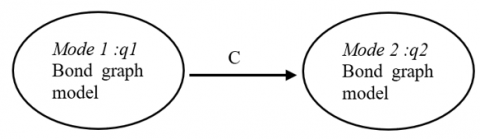Figure 1. Bond graph-hybrid automaton coupling

4. Fault Detection and Isolation Method

4.1 Model error diagnosis

A model containing errors leads directly to a wrong diagnosis. Thanks to the notion of causality, bond graph detects preliminary modeling errors and provides a first diagnostic layer.

In bond graphs, the inputs and outputs are organized by the effort causality and flow causality. The causality assignment process categorizes efforts and flows into input and output sets. It establishes the cause and effect relationships between power factors and allows the detection of modeling inconsistencies [25, 26].

Table 1 below lists the fundamental causal constraint of bond graphs .

Table 1. Bond graph’s basic multi-ports elements

Multi-port name

Power flow

Computational causality

Effort source

## t1.png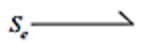## r1.png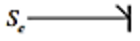Flow source

## t2.png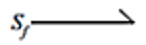## r2.png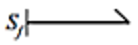Resistor

## t3.png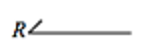## r3.png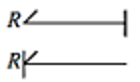Capacitor

## t4.png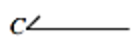## r4.png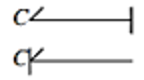Inertia

## t5.png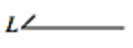## r5.png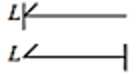Transformer

## t6.png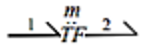## r6.png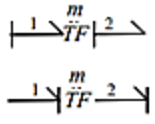Gyrator

## t7.png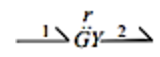## r7.png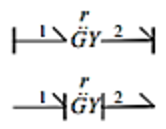0-junction

## t8.png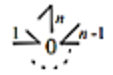## r8.png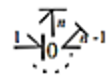1-junction

## t9.png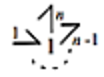## r9.png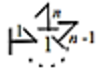The column, computational causality, in Table 1 presents the possible causality of each element. The causal stroke, at one end of a bond specifies the direction of the effort, while the flow is always in the opposite direction as shown in Figure 2 below:

## 2.png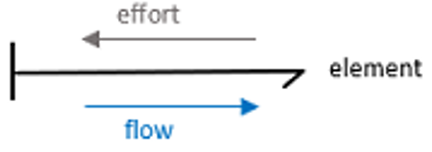Figure 2. Causal stroke of an element

Having an inconsistency of causality rules reveals a modeling error that must be rectified before starting the fault detection and isolation adventure.

4.2 Fault detection logic

Consider a nonlinear dynamical system that has m outputs and functions in n modes. The state-space represented in Eq. (1) describes the linear dynamical behavior of a mode i $\in$ [1,n].

$S_{i}:\left\{\begin{array}{l}\dot{x}(t)=A_{i} . x(t)+B_{i} . u(t)+E_{i x} . d(t) \\ y(t)=C_{i} . x(t)+D_{i} . u(t)+E_{i y} . d(t)\end{array}\right.$    (1)

The approach presented in this paper concerns only sensors faults; as a result, we consider that $E_{i x}=0$, and the system is described by Eq. (2):

$S_{i}:\left\{\begin{array}{c}\dot{x}(t)=A_{i} . x(t)+B_{i} . u(t) \\ y(t)=C_{i} . x(t)+D_{i} . u(t)+E_{i} . d(t)\end{array}\right.$    (2)

where, $x(t) \in \mathbb{R}^{k}$ is the state vector, $y(t) \in \mathbb{R}^{m}$ is the output vector, $u(t) \in \mathbb{R}^{p}$ is the input vector, $d(t) \in \mathbb{R}^{k}$ represents the vector of defaults.

The system matrix is $A_{i} \in \mathbb{R}^{k * k}$, the control matrix is $B_{i} \in \mathbb{R}^{k * p}$, the output matrix is $C_{i} \in \mathbb{R}^{m * k}$ the feedthrough matrix is $D_{i} \in \mathbb{R}^{m * p}$.

$E_{i} \in \mathbb{R}^{m * k}$ is the defect distribution matrix acting on the measurement equation (faults sensors) in a mode i.

Each mode is described by Eq. (3):

$S_{i j}:\left\{\begin{array}{c}\dot{x}(t)=A_{i} . x(t)+B_{i} . u(t) \\ y_{j}(t)=C_{i j} . x(t)+D_{i j} . u(t)+E_{i j} . d(t)\end{array}\right.$    (3)

where,  $i \in$ [1,n] and j$\in$ [1,m].

We associate to each output j evolving in a mode i an observer. Luenberger Observer is a simple and robust observer that can be used to applicate our method. It is represented by the mathematical model in Eq. (4):

$O_{i j}:\left\{\begin{array}{c}\check{\dot{x}}(t)=A_{i}. \check{x}(t)+B_{i} . u(t)+L_{i j}(y(t)-\check{y}(t)) \\ \check{y_{j}}(t)=C_{i j}. \check{x}(t)+D_{i j} . u(t) \\ \check{x}(0)=\check{x}_{0}\end{array}\right.$    (4)

As shown in Eq. (5), $e_{i j}(t)$ is the estimation error between the system state x (t) and the reconstructed state $\check{x}(t)$:

$e_{i j}(t)=x(t)-\check{x}(t)$    (5)

As shown in Eq. (6), the residue $r_{i j}(t)$ is the estimation error between the obtained output $y(t)$ and the reconstructed output $\breve{y}(t)$:

$r_{i j}(t)=y(t)-\breve{y}(t)$    (6)

The error derivative gives the following results in Eq. (7):

$\left\{\begin{array}{c}\dot{e}_{i j}(t)=\left(A_{i}-L_{i j} C_{i j}\right) . e_{i j}(t)-L_{i j} E_{i j} d(t) \\ \dot{r}_{i j}(t)=C_{i j} . e_{i}(t)+E_{i j} . d(t)\end{array}\right.$    (7)

## 3.png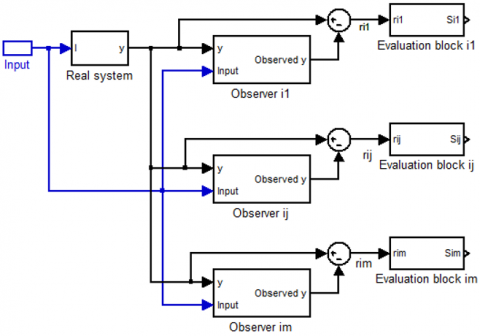Figure 3. Observer's structure

As shown in Figure 3, each observer receives all the inputs outputs of the system. Reconstructed outputs $\breve{y}(t)$ are compared instantly to measured outputs to generate residual vectors rij.

The based residual evaluation compares each element calculated to a threshold Tij, which is defined according to the disturbances modeling errors and noise measurements; we then use the following decision logic:

If ǀrij(t)ǀ>Tij

Sij=1 the output j is faulty

Else Sij=0.

The identification of the defected output consists in making correspondence between the signature obtained and the signature shown in the Table 2 below :

Table 2. Theoretical table of signatures

 Signature $C_{1}$ $C_{j}$ $C_{m}$ Si1 1 0 0 Sij 0 1 0 Sim 0 0 1

4.3 Mode identification

For a fixed output $y_{j}$, the smallest residue $r_{i j}(t)$ corresponds to the active mode i.

In order to generate the mode of each output, the residue $r_{i j}(t)$ will then be evaluated using the algorithm below:

for j=1:m

Min =$r_{1 j}(t)$;

Sj = 1;

for j = 2:n

If $r_{i j}(t)$ < Min

Min =$r_{i j}(t)$;

Sj = i;

end

end

end

S=(Sj) $\in \mathbb{R}^{m}$

If there is no default, then S(t)=a.U.

where, a is the active mode and U is the unit vector U=$\left(\begin{array}{l}1 \\ 1 \\ \cdot\\ \cdot \\ 1\end{array}\right)$.

Else, the $S_{d}(t)$ of the defected output will be different from the others $S_{j}(t)$.

The active mode is the mode of most outputs.

We suppose that we can't have more than half of the number of sensors returning errors at the same time.

V= zeros(1,m);

for j=1:m

r=0;

for i=1:m

if $S_{i}$== $S_{j}$

r=r+1;

end

end

$V_{j}$=r;

End

$V_{j}$ is the number of repetition of $S_{j}$.The highest value, $V_{c}$ , of $V_{j}$ corresponds to the active mode.

c=1;

Max= $V_{1}$;

for k=1:m

if $V_{k}$> Max

Max=$V_{k}$;

c=k;

end

end

Sc is the mode with the higher number of repetitions of Sj.

In order to make the diagnosis more reliable, we add another constraint on $V_{c}$, which is the higher number of repetitions of $S_{j}$. If $V_{c}$>m/2, then the active mode is $S_{c}$. Else, the active mode is a default mode.

5. Application Example and Simulation

5.1 Description of the system

The switched mechatronic system chosen for the application of the diagnosis method is shown in Figure 4.

## 4.pngFigure 4. Mechatronic system example

The system is composed of a voltage source, a DC motor providing a rotational movement, and a pump transforming this latter movement to a hydraulic power in order to drive the water to the tank.

The main purpose of this system is to maintain the liquid level in the tank on a well-defined level: 0.8≤l≤1.5.

To control the water level in the tank, we act on the valve states, which can have two positions:

Closed: mode 1

Opened: mode 2

Figure 5 shows the normal states of the system represented by a hybrid automaton. To jump from a mode to another, the system has to satisfy the conditions on the continuous state.

## 5.png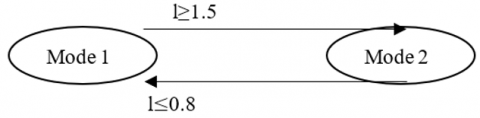Figure 5. Hybrid automaton of the system

5.2 Behavior model

In order to simplify the study and focus on the diagnosis method, the BG model of the system is built by taking into account the following suppositions:

The voltage source is constant.

The electrical part (stator winding) of the DC motor is composed of a resistance Ra and an inductance La. Its mechanical part is represented by an inertia J and a mechanical friction f.

The pump flow f is proportional to the motor rotation Velocity ωf=Cyω.

The BG model in integral causality of the mechatronic system is given in Figures 6 and 7.

For each mode, we design a bond graph model:

Mode 2:

## 6.png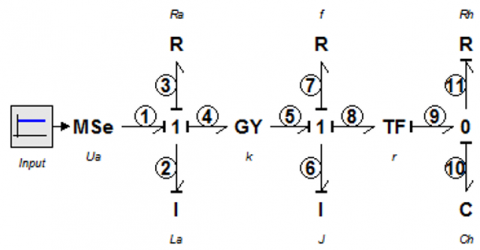Figure 6. BG model of the second mode

Mode 1:

## 7.png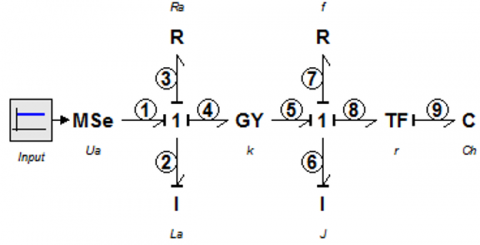Figure 7. BG model of the first mode

Bond graph in integral causality is used to generate the dynamic model of the system by following the steps below:

• Define the inputs and outputs variables
• Write the junction equations
• Write the active and passive elements equations
• Combine the above equations to get the state space equations

The equations derived from the junctions “0” and “1,” the transformer “TF,” and the gyrator “GY” of the bond graph model are given for the two modes in Eq. (8) and Eq. (15) below:

Mode 2:

$\left\{\begin{array}{c}f 1=f 2=f 3=f 4=\{i\} \\ e 1=e 2+e 3+e 4\end{array} ; \begin{array}{l}e 5=k f 4 \\ e 4=k f 5\end{array}\right.$

$\left\{\begin{array}{c}f 5=f 7=f 8=f 6=\{\omega\} \\ e 5=e 6+e 7+e 8\end{array}\right.;$

$\left\{\begin{array}{l}f 9=r . f 8 \\ e 8=r . e 9\end{array} ;\left\{\begin{array}{c}f 9=f 10+f 11 \\ e 9=e 10=e 11=\{l\}\end{array}\right.\right.$    (8)

Transformer module $r=C y . \rho . g$.

The constitutive equations of the bond graph elements are given in Eq. (9):

$e 3=R a . f 3 ; e 2=L a . \frac{d f 2}{d t} ; e 7=f . f 7 ; e 6=J . \frac{d f 6}{d t}$;

$e 11=R h . f 11 ; f 10=C h . \frac{d e 10}{d t} ; R h=\frac{R}{\rho . g} ; C h=S$    (9)

The dynamic model of the mechatronic system can be obtained in the form represented in Eq. (10) below:

$\left\{\begin{array}{l}\frac{d i}{d t}=\frac{U}{L a}-\frac{R a}{L a} i-\frac{k}{L a} \omega \\ \frac{d \omega}{d t}=\frac{k}{J} i-\frac{f}{J} \omega-\frac{C y . \rho . g}{J} l \\ \frac{d l}{d t}=\frac{C y . \rho . g}{S} \omega-\frac{1}{R h . S} l\end{array}\right.$    (10)

We can represent our system under the state space in Eq. (11):

$\left\{\begin{array}{c}\dot{X}=A_{2} . X+B_{2} . u \\ Y=C_{2} . X\end{array}\right.$    (11)

where, X=$\left(\begin{array}{c}f 1 \\ f 8 \\ e 10\end{array}\right)$= $\left(\begin{array}{c}i \\ \omega \\ l\end{array}\right)$.

The system matrix is represented by Eq. (12):

$A_{2}=\left(\begin{array}{ccc}-\frac{R a}{L a} & -\frac{k}{L a} & 0 \\ \frac{k}{J} & -\frac{f}{J} & -\frac{C y . \rho . g}{J} \\ 0 & \frac{C y . \rho . g}{S} & -\frac{1}{R h . S}\end{array}\right)$    (12)

The control matrix is represented by Eq. (13):

$B_{2}=\left(\begin{array}{c}\frac{1}{La} \\ {0} \\ 0\end{array}\right)$    (13)

The output matrix is represented by Eq. (14):

$C_{2}=\left(\begin{array}{lll}1 & 0 & 0 \\ 0 & 1 & 0 \\ 0 & 0 & 1\end{array}\right)$    (14)

Mode 1:

$\left\{\begin{array}{c}f 1=f 2=f 3=f 4=\{i\} \\ e 1=e 2+e 3+e 4\end{array} ;\left\{\begin{array}{l}e 5=k f 4 \\ e 4=k f 5\end{array}\right.\right.$

$\left\{\begin{array}{r}f 5=f 7=f 8=f 6=\{\omega\} \\ e 5=e 6+e 7+e 8\end{array} ;\left\{\begin{array}{l}f 9=r . f 8 \\ e 8=r . e 9\end{array}\right.\right.$    (15)

Transformer module r=Cy. $\rho$.g.

The constitutive equations of the bond graph elements are given in Eq. (16) below:

$e 3=R a . f 3 ; e 2=L a . \frac{d f 2}{d t} ; e 7=f . f 7$

$e 6=J . \frac{d f 6}{d t} ; f 9=C h . \frac{d e 9}{d t} ; C h=S$    (16)

The dynamic model of the mechatronic system can be obtained in the form of state space represented in Eq. (17) below:

$\left\{\begin{array}{c}\frac{d i}{d t}=\frac{U}{L a}-\frac{R a}{L a} i-\frac{k}{L a} \omega \\ \frac{d \omega}{d t}=\frac{k}{J} i-\frac{f}{J} \omega-\frac{C y . \rho . g}{J} l \\ \frac{d l}{d t}=\frac{C y . \rho . g}{S} \omega\end{array}\right.$    (17)

We can represent our system under the state space in Eq. (18) below:

$\left\{\begin{array}{c}\dot{X}=A_{1} . X+B_{1} . u \\ Y=C_{1} . X\end{array}\right.$    (18)

where, X= $\left(\begin{array}{c}f 1 \\ f 8 \\ e 10\end{array}\right)$= $\left(\begin{array}{c}i \\ \omega \\ l\end{array}\right)$.

The system matrix is represented by Eq. (19):

$A_{1}=\left(\begin{array}{ccc}-\frac{R a}{L a} & -\frac{k}{L a} & 0 \\ \frac{k}{J} & -\frac{f}{J} & -\frac{C y . \rho . g}{J} \\ 0 & \frac{C y . \rho. g}{S} & 0\end{array}\right)$    (19)

The control matrix is represented by Eq. (20):

The output matrix is represented by Eq. (21):

$B_{1}=\left(\begin{array}{c}\frac{1}{L a} \\ {0} \\ 0\end{array}\right)$    (20)

$C_{1}=\left(\begin{array}{lll}1 & 0 & 0 \\ 0 & 1 & 0 \\ 0 & 0 & 1\end{array}\right)$    (21)

5.3 Observer-based fault diagnosis method

The simplest kind of observers that we can use to applicate our method is Luenberger, which is represented by the mathematical model in Eq. (22) below:

$O_{i j}:\left\{\begin{array}{c}\check{\dot{x}}(t)=A_{i} . \check{x}(t)+B_{i} . u(t)+L_{i j}(y(t)-\breve{y}(t)) \\ \breve{y}_{j}(t)=C_{i j} . \check{x}(t)+D_{i j} . u(t) \\ \check{x}(0)=\check{x}_{0}\end{array}\right.$    (22)

In this example, the bank of observers used, consists of six observers: $O_{i j}$, i $\in${1, 2}, j $\in${1, 2, 3}. Each observer is sensitive to an output.

Let us use the pole placement technique to determine the observer gains. To do this, the poles are chosen as follows in Eq. (23):

$\mathrm{P}_{11}=\mathrm{P}_{12}=\mathrm{P}_{13}=\mathrm{P}_{21}=\mathrm{P}_{22}=\mathrm{P}_{23}=\left(\begin{array}{c}-70+\mathrm{i} \\ -70-\mathrm{i} \\ -70\end{array}\right)$    (23)

The gain of each observer is defined in Eq. (24):

$\mathrm{L}_{11}=10^{2}\left(\begin{array}{c}1.3 \\ -3701 \\ 259.3\end{array}\right) ; \mathrm{L}_{12}=\left(\begin{array}{c}-0.03 \\ 131.2 \\ -12.9\end{array}\right)$;

$\mathrm{L}_{13}=10^{2}\left(\begin{array}{c}-1.6 \\ 2512.3 \\ 1.31\end{array}\right) ; \mathrm{L}_{21}=10^{2}\left(\begin{array}{c}1.3 \\ -3645.2 \\ 248.3\end{array}\right)$;

$\mathrm{L}_{22}=\left(\begin{array}{c}-0.03 \\ 130.2 \\ -12.6\end{array}\right) ; \mathrm{L}_{23}=10^{2}\left(\begin{array}{c}-1.6 \\ 2512.3 \\ 1.3\end{array}\right)$    (24)

The next step is the construction of a bond graph model equivalent to the observer equations.

This model, noted OBG, is composed of the term $L(Y-\check{Y})$ added to the IBG model.

Figures 8 and 9 represent the linear injection of the term $L(Y-\check{Y})$ into the dynamic elements I and C, using modulated sources: the modulated flow source MSf for an element I and the modulated effort source MSe for an element C.

The “E” used below is a symbolic representation of an element to which C or I is attached by a bond.

## 8.pngFigure 8. The case of the element C

## 9.png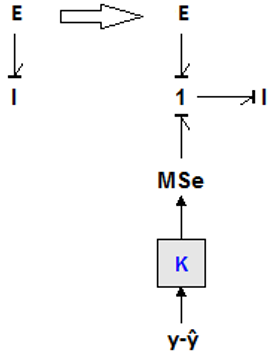Figure 9. The case of the element I

The observer BG model of each mode is shown in Figure 10 and 11 below:

K1, K2, K3 are the components of the vector $L_{i j}$, i $\in$ {1, 2}, j $\in$ {1, 2, 3}.

Mode 2

## 10.png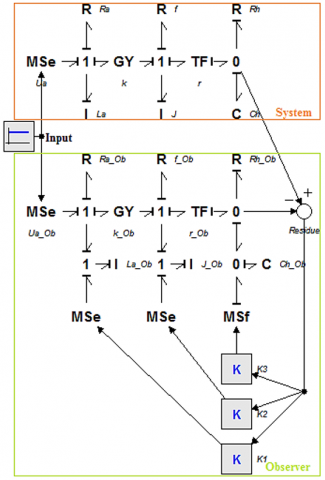Figure 10. BG model of the second mode and its observer

Mode 1

## 11.png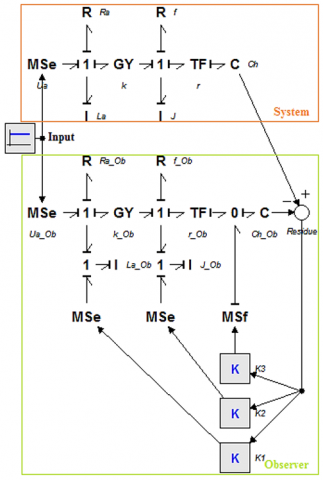Figure 11. BG model of the first mode and its observer

5.4 Simulation results

The simulation of the system has been performed by the software Matlab and 20-sim. The numerical values of parameters are shown in Table 3.

Table 3. Numerical values of parameters

 Symbol Description Numerical value Ua Voltage source 48 V Ra Electrical resistance of the DC motor 4 Ω La Inductance of the DC motor 55 mH J Inertia of the rotation part $43.10^{-6} \mathrm{Kg} . \mathrm{m}^{2}$ f Friction coefficient of the mechanical part of the DC motor $26.10^{-5} \mathrm{~N} . \mathrm{m} . \mathrm{s}$ k Gyrator ratio 0.002 V.S/rad g gravitational acceleration $9.8 \mathrm{~m} . \mathrm{s}^{-2}$ S Surface area of the base of the tank $1 \mathrm{~m}^{2}$ Cy Volumetric displacement of hydraulic pump $1.59 .10^{-6} \mathrm{~m}^{3} / \mathrm{rad}$ R Hydraulic resistance of the valve $9.8 .10^{3}$ N.s. $\mathrm{m}^{-5}$ $\rho$ Water Density $10^{3} \mathrm{Kg} . \mathrm{m}^{-3}$

The normal evolution of the discrete and the continuous states are presented in Figure 12. The switching between modes occurs when the conditions are realized. The Simulation time is fixed at 10s to show the dynamic behavior of the system in the two modes.

The graphs above give a view on the evolution of the system parameters. Each mode is distinguished from the other by a different dynamic.

The zoom on the level l of the tank in Figure 13 shows a water level varying between 0.8 and 1.5 and respecting the preliminary conditions.

In order to test the effectiveness of our proposed diagnosis approach, we inject a sensor fault and we present the response of the system. Our aim is to detect the active mode and locate the defected output.

## 12.png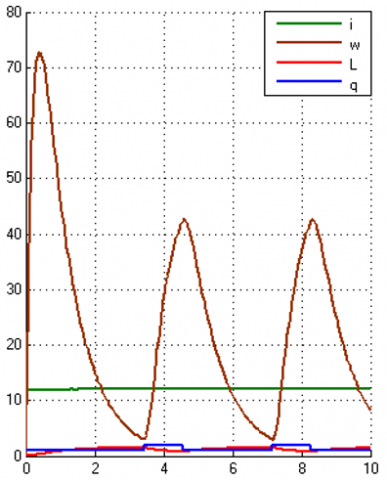Figure 12. The discrete-continuous evolution of the system

## 13.png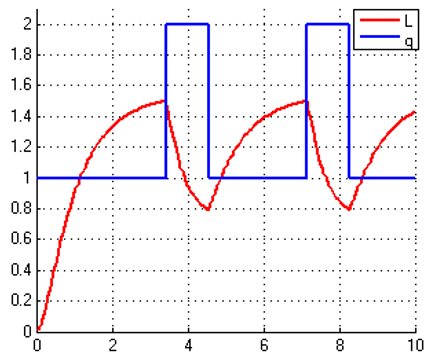Figure 13. Zoom on the level l of the tank

## 14.png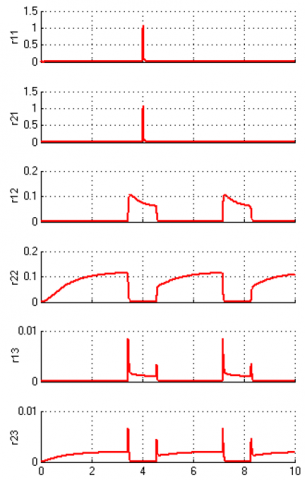Figure 14. Residues

Figure 14 shows the residues responses. The fault detection decision consists in comparing the residues to defined thresholds.

The detection thresholds of the chosen mechatronic system are given in the Table 4.

Table 4. Detection thresholds

 Threshold T11 T21 T12 T22 T13 T23 Numerical value 0.03 0.03 0.1 0.1 0.03 0.03

We notice that at the instant 4s, the two residues r11 and r21 depart significantly from zero and exceed their respective threshold T11 and T2,1 which means the detection of an error in the first output y1.

Figure 15 shows the result of the residues evaluation generating the sensors signature below:

## 15.png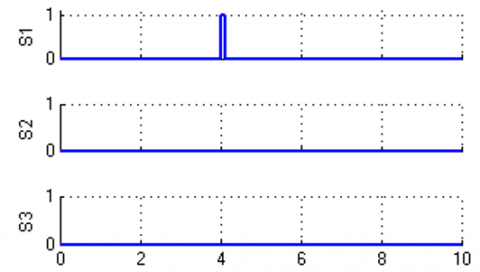Figure 15. Sensor signature

Using sensors signatures, the fault is clearly detected. The next step is the identification of the active mode.

Figure 16 shows the generated signatures corresponding to the mode of each output.

## 16.png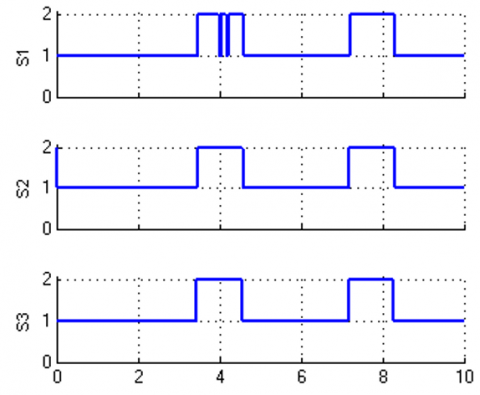Figure 16. Mode signature

Our algorithm detects the active mode using feedback from mode signatures. Figure 17 shows the comparison between the real modes from the system and the estimation modes from observers.

We note that the real, and the estimation modes are the same.

The application of the proposed method on the chosen example has given good results. It has been able to detect and locate the defect in a reliable and instantaneous way, and it has proved that it deserves to be used and deployed in the industrial sector.

## 17.png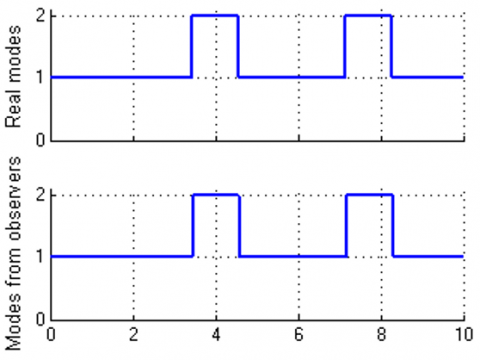Figure 17. Real modes VS estimated modes

6. Conclusion

This paper proposes a hybrid fault diagnosis method applied to switched mechatronic systems. It is a new model-based approach allowing fault detection and isolation of several industrial systems. The first phase concerns the construction of the system model using Bond Graph-Hybrid Automaton Coupling, where the continuous state is modeled by a bond graph and the discrete state is described by a hybrid automaton.

A first model diagnosis layer is detailed to detect modeling errors using bond graphs, and a second fault detection and isolation method is elaborated to detect and locate faults using hybrid observers.

The focus has always been on mechatronic systems independently of hybrid systems or vice versa. The advantage of this method is its ability to detect sensor faults in most industrial systems. Whether for mechatronic systems, switching systems, or both, the proposed approach finds a fast and powerful solution by optimizing the number of calculations.

The present study has only examined sensor faults. Therefore, an extension of the proposed method to the diagnosis of actuators could be a relevant perspective to enlarge the approach and make it more efficient.

References

 Chiang, L.H., Russell, E.L., Braatz, R.D. (2000). Fault Detection and Diagnosis in Industrial Systems. Springer Science & Business Media.

 Doraiswami, R., Cheded, L., Demetgul, M., Ünal, M. (2017). Fault Detection and Isolation. In Fault Diagnosis and Detection. InTech.

 Isermann, R. (1990). Estimation of physical parameters for dynamic processes with application to an industrial robot. 1990 American Control Conference, San Diego, CA, USA, pp. 1396-1401. https://doi.org/10.23919/ACC.1990.4790968

 Patton, R.J., Chen, J. (1991). A review of parity space approaches to fault diagnosis. IFAC Proceedings Volumes, 24(6): 65-81. https://doi.org/10.1016/S1474-6670(17)51124-6

 Frank, P.M. (1987). Advanced fault detection and isolation schemes using nonlinear and robust observers. IFAC Proceedings Volumes, 20(5): 63-68. https://doi.org/10.1016/S1474-6670(17)55353-7

 Hammouri, H., Kinnaert, M., El Yaagoubi, E.H. (1999). Observer-based approach to fault detection and isolation for nonlinear systems. IEEE Transactions on Automatic Control, 44(10): 1879-1884. https://doi.org/10.1109/9.793728

 Najeh, T., Njima, C.B., Garna, T., Ragot, J. (2017). Input fault detection and estimation using PI observer based on the ARX-Laguerre model. The International Journal of Advanced Manufacturing Technology, 90(5): 1317-1336. https://doi.org/10.1007/s00170-016-9414-6

 Balluchi, A., Benvenuti, L., Di Benedetto, M.D., Sangiovanni-Vincentelli, A.L. (2002). Design of observers for hybrid systems. In International Workshop on Hybrid Systems: Computation and Control, Stanford, CA, USA, pp. 76-89. https://doi.org/10.1007/3-540-45873-5_9

 Abboudi, A., Belmajdoub, F. (2020). A new diagnosis approach of hybrid systems through Observers and hybrid automata. International Journal of Automation and Smart Technology, 10(1): 135-143. https://doi.org/10.5875/ausmt.v10i1.2169

 Borutzky, W. (2015). Bond Graph Model-Based Fault Diagnosis of Hybrid Systems. Cham: Springer International Publishing.

 Kazemi, M.G., Montazeri, M. (2019). Fault detection of continuous time linear switched systems using combination of bond graph method and switching observer. ISA Transactions, 94: 338-351. https://doi.org/10.1016/j.isatra.2019.04.023

 Carryer, J.E., Ohline, R.M., Kenny, T.W. (2011). Introduction to Mechatronic Design. Prentice Hall. https://books.google.es/books/about/Introduction_to_Mechatronic_Design.html?id=Wcc7PQAACAAJ&source=kp_book_description&redir_esc=y.

 Isermann, R. (2007). Mechatronic Systems: Fundamentals. Springer Science & Business Media.

 Liberzon, D. (2003). Switching in Systems and Control. Springer Science & Business Media.

 Liberzon, D. (2005). Switched systems. in Handbook of Networked and Embedded Control Systems, Springer, 559‑574. https://doi.org/10.1007/0-8176-4404-0_24

 Paynter, H.M. (1992). An epistemic prehistory of bond graphs. Bond Graphs Eng., 3‑17.

 Rosenberg, R.C., Karnopp, D.C. (1972). A definition of the bond graph language. Journal of Dynamic Systems, Measurement, and Control, 94(3): 179‑182. https://doi.org/10.1115/1.3426586

 Samantaray, A.K., Medjaher, K., Bouamama, B.O., Staroswiecki, M., Dauphin-Tanguy, G. (2006). Diagnostic bond graphs for online fault detection and isolation. Simulation Modelling Practice and Theory, 14(3): 237-262. https://doi.org/10.1016/j.simpat.2005.05.003

 Samantaray, A.K., Bouamama, B.O. (2008). Bond graph modeling in process engineering. Model-Based Process Supervision: A Bond Graph Approach, pp. 13-80. https://doi.org/10.1007/978-1-84800-159-6

 Said, M., Fazai, R., Abdellafou, K.B., Taouali, O. (2018). Decentralized fault detection and isolation using bond graph and PCA methods. The International Journal of Advanced Manufacturing Technology, 99(1): 517-529. https://doi.org/10.1007/s00170-018-2526-4

 Medjaher, K., Zerhouni, N. (2013). Hybrid prognostic method applied to mechatronic systems. The International Journal of Advanced Manufacturing Technology, 69(1-4): 823-834. https://doi.org/10.1007/s00170-013-5064-0

 Gawthrop, P.J., Bevan, G.P. (2007). A tutorial introduction for control engineers. IEEE Control Systems, 27(2): 24-45. https://doi.org/10.1109/MCS.2007.338279

 Henzinger, T.A. (2000). The theory of hybrid automata. In Verification of Digital and Hybrid Systems, pp. 265-292. https://doi.org/10.1007/978-3-642-59615-5_13

 Ezzahra, O.F., Zanzouri, N. (2015). Hybrid dynamical system monitoring based on bond graph. 3rd International Conference on Automation, Control Engineering & Computer Science, pp. 332-337.

 Borutzky, W. (2010). Analysis of causal bond graph models. Bond Graph Methodology: Development and Analysis of Multidisciplinary Dynamic System Models, pp. 223-303. https://doi.org/10.1007/978-1-84882-882-7_6

 Behzadipour, S., Khajepour, A. (2006). Causality in vector bond graphs and its application to modeling of multi-body dynamic systems. Simulation Modelling Practice and Theory, 14(3): 279-295. https://doi.org/10.1016/j.simpat.2005.06.001

 Wong, T., Cormier, G. (2007). Bond graph causality assignment and evolutionary multi-objective optimization. In Advances and Innovations in Systems, Computing Sciences and Software Engineering, pp. 433-438. https://doi.org/10.1007/978-1-4020-6264-3_75